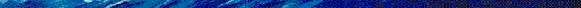## Math 220A Lecture NotesDescription PDF File
Introduction intro.pdf
First-Order Equations firstorder.pdf
Conservation Laws conservation.pdf
Classification of Second-Order Equations secondorder.pdf
Wave Equation On R waveequation1.pdf
Wave Equation on an Interval waveequation2.pdf
Wave Equation in Higher Dimensions waveequation3.pdf
Hyperbolic Systems of First-Order Equations systems.pdf